## Calculating the Vegetation Indices from Landsat 8 image Using ArcGis 10.1

The Normalized Differential Vegetation Index (NDVI) is a standardized vegetation index which allows us to generate an image showing the relative biomass. The chlorophyll absorption in Red band and relatively high reflectance of vegetation in Near Infrared band (NIR) are using for calculating NDVI. ( From ESRI)

“An NDVI is often used worldwide to monitor drought, monitor and predict agricultural production, assist in predicting hazardous fire zones, and map desert encroachment. The NDVI is preferred for global vegetation monitoring because it helps to compensate for changing illumination conditions, surface slope, aspect, and other extraneous factors” (Lillesand 2004).

Monitoring the intensity and the density of the green vegetation growth can be done using the reflection from the red band and the infrared band. Green vegetation reflects more energy in the near- infrared band than in the visible range. It observe red band more for the photosynthesis process. Leaves reflects less in the near-infrared region when they are stressed, diseased or dead. Features like Clouds, water and snow show better reflection in the visible range then the near-infrared range, while the difference is almost zero for rock and bare soil.

Output of the NDVI method creates a single-band dataset that only shows greenery. Values close to zero represent rock and bare soil and negative values represent water, snow and clouds. Taking ratio or difference of two bands makes the vegetation growth signal differentiated from the the background signal. NDVI method was developed by the NASA scientist popularly known as Normalized Difference Vegetation Index (NDVI). By taking a ratio of two bands drop the values between -1 to +1. Table below shows the red and infrared bands reflectance values of features and their NDVI values. Water has an NDVI value less than 0, bare soils between 0 and 0.1, and vegetation over 0.1. Increase in the positive NDVI value means greener the vegetation.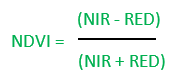RED= DN values from RED band

NIR= DN values from Near Infrared band

 COVER TYPE RED NIR NDVI Dense vegetation 0.1 0.5 0.7 Dry Bare soil 0.269 0.283 0.025 Clouds 0.227 0.228 0.002 Snow and ice 0.375 0.342 -0.046 Water 0.022 0.013 -0.257

Fig: Table 1

Table shows typical reflectance values in the red and infrared channels, and the NDVI for typical cover types. Water typically has an NDVI value less than 0, bare soils between 0 and 0.1 and vegetation over 0.1.

Here we are using the Landsat image acquired from USGS Earth Explorer. The data is in GeoTiff format with 16 bit radiometric resolution (ranges from 0-65535). Landsat 8 Operational Land Imager (OLI) and Thermal Infrared Sensor (TIRS) images consist of nine spectral bands with a spatial resolution of 30 meters for Bands 1 to 7 and 9. The resolution for Band 8 (panchromatic) is 15 meters. In addition it also have two Thermal IR bands with a spatial resolution of 100m (later resampled into 30 m).Before calculating the NDVI the DN data must be converted to reflectance using the equations given in their website. Here the IR and NIR bands are 4 and 5 respectively.

Table shows the Spectral characteristics of Landsat 8

 Landsat 8 Operational Land Imager (OLI) and Thermal Infrared Sensor (TIRS) Bands Wavelength Resolution Band 1 – Coastal aerosol 0.43 – 0.45 30 Band 2 – Blue 0.45 – 0.51 30 Band 3 – Green 0.53 – 0.59 30 Band 4 – Red 0.64 – 0.67 30 Band 5 – Near Infrared (NIR) 0.85 – 0.88 30 Band 6 – SWIR 1 1.57 – 1.65 30 Band 7 – SWIR 2 2.11 – 2.29 30 Band 8 – Panchromatic 0.50 – 0.68 15 Band 9 – Cirrus 1.36 – 1.38 30 Band 10 – Thermal Infrared (TIRS) 1 10.60 – 11.19 100 * (30) Band 11 – Thermal Infrared (TIRS) 2 11.50 – 12.51 100 * (30)

(Obtained from USGS website)

## Step 1: Calculating Reflectance value from the Satellite data

OLI spectral radiance data can also be converted to TOA planetary reflectance using reflectance rescaling coefficients provided in the landsat8 OLI metadata file. The following equation is used to convert DN values to TOA reflectance for OLI image:

ρλ’ = M ρQ cal + A ρ

Where:

ρλ’ = TOA planetary reflectance, without correction for solar angle. Note that ρλ’ does not contain a correction for the sun angle.

M ρ = Band-specific multiplicative rescaling factor from the metadata (Reflectance_Mult_Band_x, where x is the band number)

A ρ = Band-specific additive rescaling factor from the metadata (Reflectance_Add_Band_x, where x is the band number)

Q cal = Quantized and calibrated standard product pixel values (DN).

(Metadata means the .txt file you can find along with the data)

I. Open ArcMap and add satellite images band 4 and band 5 for calculating NDVI.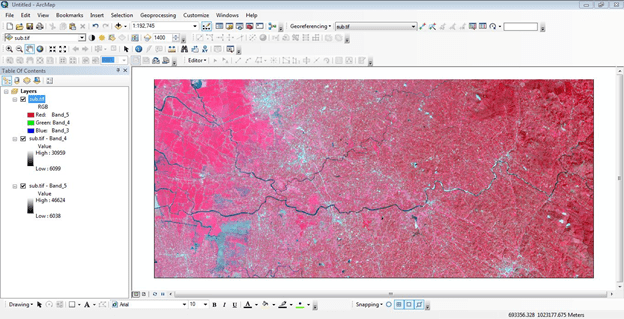II. Open Arc toolbox in ArcMap

II. From the Arc toolbox open Raster calculator (Arc toolbox >Spatial Analyst tool > Map Algebra tool > Raster Calculator tool)IV. Find M ρ Band-specific multiplicative rescaling factor from the metadata    (Reflectance_Mult_Band_x, where x is the band number)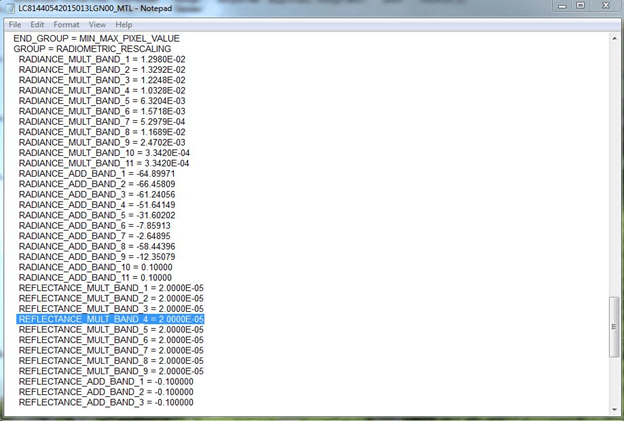An example of where the M ρ Band-specific multiplicative rescaling factor from the metadata

(Reflectance_Mult_Band_x, where x is the band number) is found within the metadata file

Apply and perform values in Raster calculator for both RED and NIR bands (4 and 5 bands)

Example

Band 4 reflectance= (2.0000E-05 * (“sub_tif_Band_4”)) + -0.100000

Where

REFLECTANCE_MULT_BAND_4 = 2.0000E-05

sub_tif_Band_4= 4th band (Red band)

Set the output folder and file name then click “OK”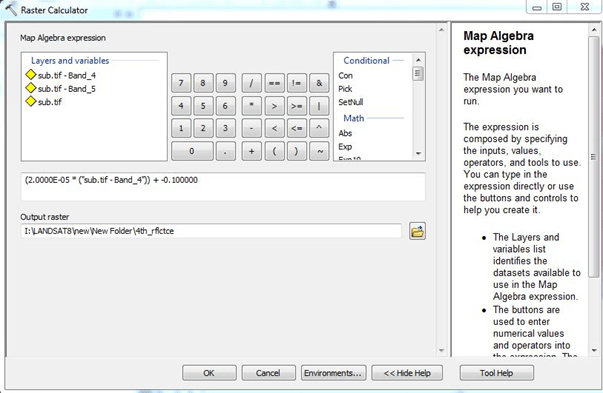Raster Calculator function to perform reflectance calculation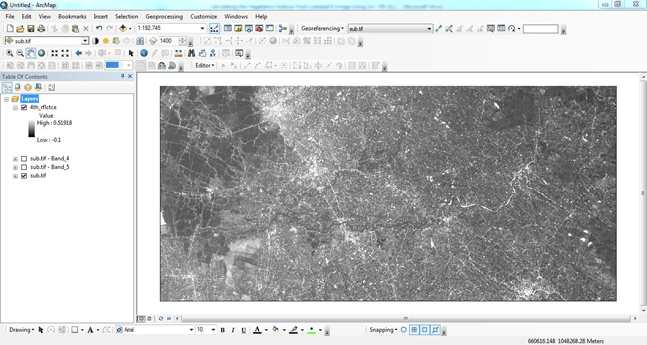Reflectance calculated for band 4

V. Calculate the Reflectance of band 5th by using the same method.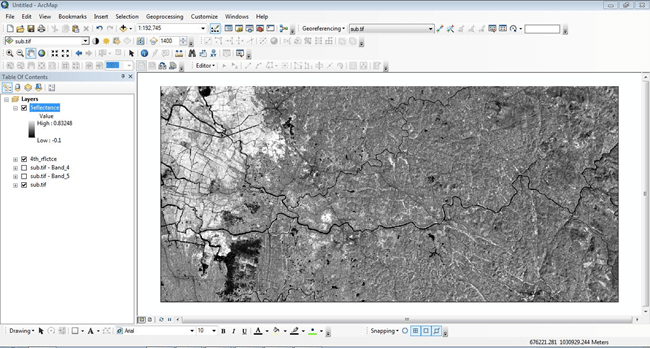Reflectance calculated for band 5

## Step 2: Correcting the Reflectance value with sun angle

Reflectance with a correction for the sun angle is then:

ρλ = ρλ ‘/cos θSZ = ρλ ‘/sin θSE

Where:

ρλ = TOA planetary reflectance

θSE = Local sun elevation angle. The scene center sun elevation angle in degrees is provided in the metadata (Sun Elevation).

θSZ = Local solar zenith angle; θSZ = 90° – θSE.

I. Find Sun Elevation from the metadata

Apply and perform the values in Raster calculator for both RED and NIR band reflectance values (4 and 5 bands)

Example

Band 4 corrected_ reflectance= (“4th_rflctnce”) / Sin (49.36816761)

Where:

SUN_ELEVATION = 49.36816761

4th_rflctce =uncorrected reflectance

Set the output folder and file name then click “OK”Raster Calculator function to perform sun angle corrected reflectanceSun angle corrected reflectance for band 4th

II. Calculate the Reflectance of band 5th by using the same methodSun angle corrected reflectance for band 5th

## Step 3: Calculating NDVI from 4th and 5th bands

As we discussed earlier to find NDVI we use the formula ofHere band 4= RED and band 5=NIR

I. Open Raster calculator and apply this formula and execute the program as follows

NDVI= (Band 5 correctedBand 4 corrected)/ (Band 5 corrected + Band 4 corrected)

Set the output folder and file name then click “OK”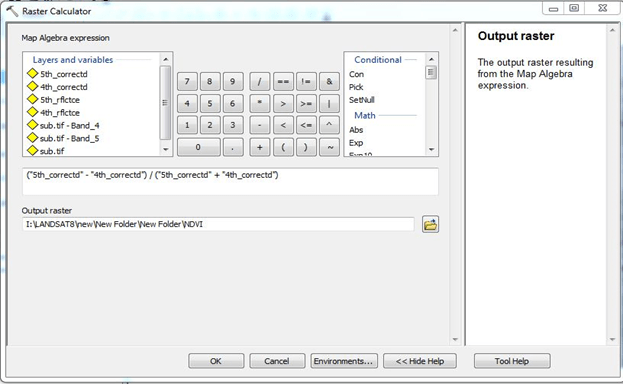Raster Calculator function to perform NDVINormalized Differential Vegetation Index

The value of NDVI is always between 1 and -1

## Step 4: Calculating SAVI from Reflectance

Soil Adjusted Vegetation Index shows background soil conditions. SAVI is a hybrid between NDVI and PVI (Perpendicular Vegetation Index).Where NIR is the 5th band and RED is the 4th band respectively. L is the Soil brightness correction factor. The value of L varies by cover of green vegetation. High vegetation areas L= 0; the areas have no green vegetation then L=1. Generally L=0.5 in most causes and it works well. When L=0 then NDVI=SAVI

I. Open Raster calculator and apply this formula and execute the program as follows

SAVI= (Band 5 correctedBand 4 corrected)/ (Band 5 corrected + Band 4 corrected)*(1+L)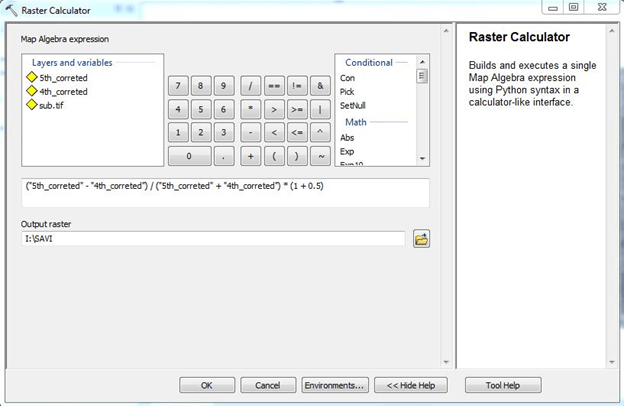Raster Calculator function to perform SAVI## Step 5: Calculating Tasseled cap indices

Tasseled cap indices give the measure of Greenness, Brightness and Wetness of each pixel and utilize a linear combination of 6 Landsat bands (From 2nd band to 7th band). Tasseled cap indices is calculated by the following equation

Tas_cap =     (coeff * band2) + (coeff₃ * band3) + (coeff₄ * band4) +

(coeff₅ * band5) + (coeff₆* band6) + (coeff₇ * band7)

Where Tas_cap is the calculated tasseled cap index for brightness, greenness, or wetness from the coefficient given

 Index Band 2 (Blue) Band 3 (Green) Band 4 (Red) Band 5 (NIR) Band 6 (SWIR 1) Band 7 (SWIR 2) Brightness 0.3029 0.2786 0.4733 0.5599 0.508 0.1872 Greenness −0.2941 −0.243 −0.5424 0.7276 0.0713 −0.1608 Wetness 0.1511 0.1973 0.3283 0.3407 −0.7117 −0.4559

Given by Muhammad Hasan Ali Baig et al (2014)

## Step 6: Calculating Tasseled cap Brightness indices

I. Calculate the Reflectance of Band 2, 3, 4, 5, 6, 7, using the same method mentioned above (Process 1 and 2)

II. Open Raster calculator and apply the formula for Brightness index and execute the program as follows

Tas_cap = (0.3029 *(2nd_Band_correted))+

( 0.2786 *(3rd_Band_correted))+

( 0.4733 *(4th_Band_correted))+

( 0.5599 *(5th_Band_correted))+

( 0.508 *(6th_Band_correted))+

( 0.1872 *(7th_Band_correted))

Raster Calculator function to perform SAVI Raster Calculator function to perform SAVIRaster Calculator function to perform Brightness index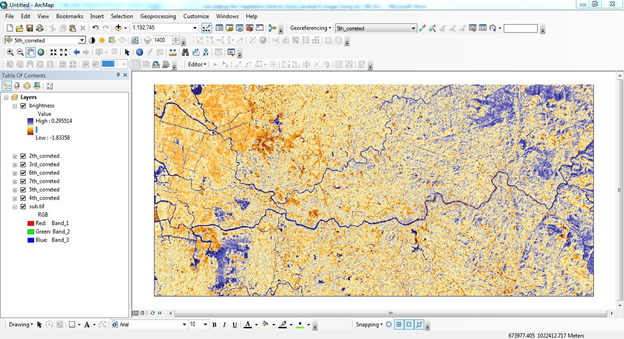Tasseled cap brightness index

## Step 7: Similarly calculate the other indices using the same method mentioned above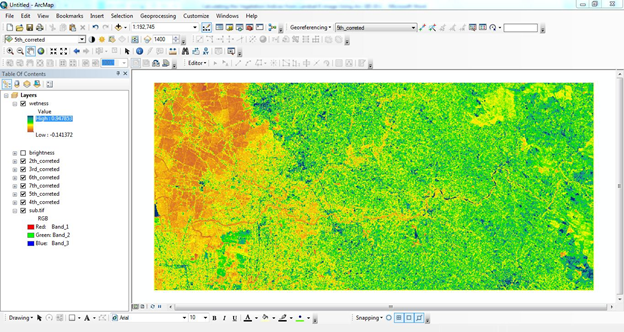Tasseled cap wetness indexTasseled cap Greenness index

References:

http://landsat.gsfc.nasa.gov/?p=3186

http://hydrology1.nmsu.edu/teaching/soil698/greenseeker/what_is_ndvi.htm

http://www.pvts.net/pdfs/ndvi/3_3_ndvi.PDF

http://wiki.landscapetoolbox.org/doku.php/remote_sensing_methods:normalized_difference_vegetation_index

Muhammad Hasan Ali Baigab, Lifu Zhanga, Tong Shuaiab & Qingxi

Tonga- “Derivation of a tasselled cap transformation based on Landsat 8 atsatellite reflectance” (2014) Remote Sensing Letters

http://www.tandfonline.com/loi/trsl20

Grant J. Firl, Lane Carter” Calculating Vegetation Indices from Landsat 5 TM and Landsat 7 ETM+ Data”(2011)

### This Post Has 36 Comments

1.hi Mate

Thank you very much for your useful information about NDVI, I have a question:
I download Landsat 8 images from USGS, mostly surface reflectance and in some case NDVI data, I want to change the resolution of NDVI to 15m, I do with resampling in Arcgis, it does and you get the 15m, but for presentation purpose on screen the sell size remain 30 m,also I did raster to point from my NDVI and then with interpolation I made 15m sell size. but this process may slightly change the NDVI data.

can I apply pan sharpening process on NDVI which is one band image? I would appreciate if you consider my questions.

kind regards
Hamid

1.I will try to find answer for you, thank you for your comment

2.Excellent article ! Congrats !

1.Thank you

3.Hello Sir…
I have calculated NDVI and SAVI for landsat-8 image as you given. But Iam getting higher value of NDVI than SAVI
Can you tell me reason for this
NDVI=0.46
SAVI=0.34
Specific band values are
toa b4- 0.130
toa b5- 0.3650

4.Hey, you’ve a mistake with corrected reflectance

http://landsat.usgs.gov/Landsat8_Using_Product.php

1.Thanks I will go through it and amend it, Appreciate for that.

5.very good your website, thank you

1.Thank you

6.Hi,
Shouldn’t you convert the angle to radians?
I thought that ArcGIS prefers to work in radians.
Also, woking with trig also requires setting the cell size and extent in the ArcGIS geoprocessing environment, something I learnt the hard way.

1.Yes you have to convert to radians in Arcgis, good info.

7.So just use:

(“4th_rflctce”) / 0.75890963 OR (“4th_rflctce”) / Sin(49.36816761 * math.pi / 180)

in the raster calculator step 1, agreed?

8.please how do you convert to radians and what do you set the cell side and extent to? please I would appreciate an immediate reply. thank you

9.can you upload the tutorial for enhanced difference vegetation index, generalized difference vegetation index, normalized difference water index, specific leaf area vegetation index, and simple ratio.

10.I have tried these steps several times using radian and degree as well,but I am getting NDVI more than +1 to -1..It is showing NDVI values from 549 to -99..How is this possible??

1.i have also same problem.. why??

11.Thank you so much. Your post gave me an easy and clear way to calculate NDVI. But I have some questions confused me a long time.
In http://earthexplorer.usgs.gov/ website, there are two different directories “L8 OLI/TIRS” and “Landsat Surface Reflectance – L8 OLI/TIRS”, what’s the difference between them? It seem that your method is used the former data. How I can calculate NDVI use the later data?
For the same pixel, the DN values of them are not identical. Could you give me some explanation for this?
For an example, LC80420352015194LGN00 :
B1 10267 sr_band1 194
B2 9326 sr_band2 259
B3 8666 sr_band3 486
B4 7922 sr_band4 452
B5 19322 sr_band5 3123
B6 13520 sr_band6 1928
B7 9597 sr_band7 1102
B8 8321 sr_cloud 16
B9 5062
B10 29159
B11 26862
BQA 20512

Thanks again.

12.For the first step :

1. Calculating Reflectance value from the Satellite data

The formula is like this Lλ = MLQcal + AL or ρλ’ = M ρQ cal + A ρ

where:

ML = Band-specific multiplicative rescaling factor from the metadata (RADIANCE_MULT_BAND_x, where x is the band number)
Qcal = Quantized and calibrated standard product pixel values (DN

so in the map algebra :

(1.0050E-02*(“LC81190652016289LGN00_B4.TIF”))+-50.24808

13.I cant run for the formula greenness and wetness and there is an error for the formulation I use as below:

Greenness
– 0.2941 *(band2corrected.img)- 0.243 *(band3corrected.img)- 0.5424 *(band4corrected.img)+(0.7276 *(band5corrected.img))+(0.0713 *(band6corrected.img))- 0.1608 *(band7corrected.img)

Wetness
(0.1511 *(band2corrected.img))+ (0.1973 *(band3corrected.img))+(0.3283 *(band4corrected.img))+(0.3407 *(band5corrected.img))+(−0.7117 *(band6corrected.img))+(−0.4559 *(band7corrected.img))

14.Please can you tell me how can i calculate Aerosol optical depth using landsat_8 data ?
thank you so much, anything would be helpful and apreciated

15.hi everyone, please tell me .1 “why we are using calculate only PM10 using landsat 8 images,why cant other(03,N02,CO2 CONCENTRATION) ?”, and also 2 “how we are calculate PM10 using landsat 8 (images, DN values and MTL files ) briefly ?”

16.Hello, Thank you for this very good explination!

I am also using Landsat 7 data for NDVI calculation and was wondering whether to do the same steps as for Landsat 8 (step 1 & 2 for correction, and then step 3 for NDVI but with band 3+4 instead of 4+5).

If this is so, where do I find Reflectance_Mult_Band_x and Reflectance_Add_Band_x for Landsat 7?

17.How can we Calculate the file size (in bit) of the Landsat 8 OLI file assuming that only the information for each pixel are stored within the image

18.i just read this, it is really helpful and systematically outlined, bravo to the author.

1.Thank you Johnie

19.Hi, congrats on the blog and the guide. I just have one question – aren’t TOA reflectance values supposed to be converted to BOA (bottom of the atmosphere) reflectance through Dark Object Subtraction, or any other method, in order to remove the atmospheric signal. As far as I know, this should be done before any kind of analysis involving indexes or classification is concerned.

1.I will see and reply to you.

20.I have done the same as you have shown, but I’m getting very higher level NDVI data why is it so ? The data is L8 OLI/TIRS

21.Sir.
how can i retrieve LAI leaf area index using NDVI and SAVI?

22.Sir,
Thank you for the guide & it was very helpful to me. As well as I need to know about the cloud correction for Landsat8, Landsat7 & Landsat5.

Thank You!

23.I liked it.

1.Thank you,

24.Hi,
Thank you for this post. I hope you’re still active.
The maximum NDVI value I get is 1.51 do you know how I can correct this? Or what might have gone wrong?

25.how can i calculate Enhanced Vegetation Index for Landsat 1-3 image and Landsat 8 image? and how can i normalize the range of EVI value as it is not a normalized index using ArcGIS 10.1?

26.Without any cultivation activity, will the NDVI values be the same for two fields that are not contagious? The two fields have about 1 Deg difference.

This site uses Akismet to reduce spam. Learn how your comment data is processed.